Search

Conics: Circles: Introduction & Drawing (page 1 of 3)

Sections: Introduction & Drawing, Working with equations, Further examplesA circle is a geometrical shape, and is not of much use in algebra, since the equation of a circle isn't a function. But you may need to work with circle equations in your algebra classes.

In "primative" terms, a circle is the shape formed in the sand by driving a stick (the "center") into the sand, putting a loop of string around the center, pulling that loop taut with another stick, and dragging that second stick through the sand at the further extent of the loop of string. The resulting figure drawn in the sand is a circle.

In algebraic terms, a circle is the set (or "locus") of points (x, y) at some fixed distance r from some fixed point (h, k). The value of r is called the "radius" of the circle, and the point (h, k) is called the "center" of the circle.

The "general" equation of a circle is:

x2 + y2 + Dx + Ey + F = 0

The "center-radius" form of the equation is:

(x  h)2 + (y  k)2 = r2

...where the h and the k come from the center point (h, k) and the r2 comes from the radius value r. If the center is at the origin, so
(h, k) = (0, 0), then the equation simplifies to x2 + y2 = r2.

Note: The "general" form may be given in your book using different letters for the coefficients, but the "center-radius" form will use the same letters as shown above: The center is always denoted by "(h, k)" and the radius is always denoted by "r". Your book might also refer to something called the "standard" form of the circle equaion. Unfortunately, "standard form" has no fixed meaning that I've been able to determine, so you'll have to keep track of what your book intends by that term.

You can convert the "center-radius" form of the circle equation into the "general" form by multiplying things out and simplifying; you can convert in the other direction by completing the square.

The center-radius form of the circle equation comes directly from the Distance Formula and the definition of a circle. If the center of a circle is the point (h, k) and the radius is length r, then every point (x, y) on the circle is distance r from the point (h, k). Plugging this information into the Distance Formula (using r for the distance between the points and the center), we get: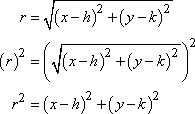You should recognize this formula; you will be expected to be able to read information from it.

• State the center and radius of the circle with the equation (x  2)2 + y2 = 52, and sketch the circle.
• The y2 term means the same thing as (y  0)2, so the equation is really (x  2)2 + (y  0)2 = 52, and the center must be at (h, k) = (2, 0). Clearly, the radius is r = 5.

 To sketch, I'll first draw the dot for the center: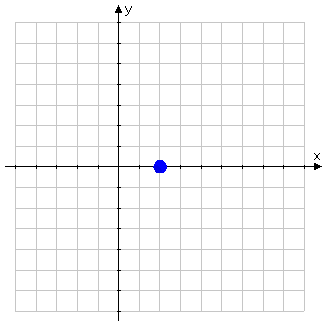Then I'll rough in markers that are five units away from the center in each of the four "easy" directions: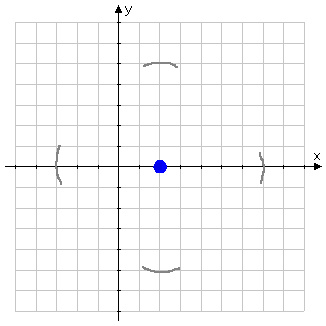Then I'll rough in the curvy bits in between these markers, turning the paper as I go:   Copyright © Elizabeth Stapel 2010-2011 All Rights Reserved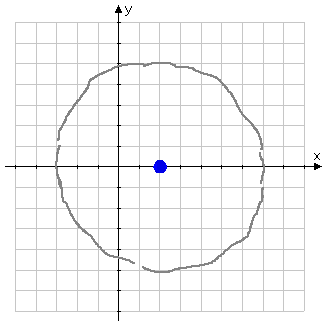I'll make whatever corrections look useful, trying to make my circle look properly circular, and draw my final answer as a solid dark line: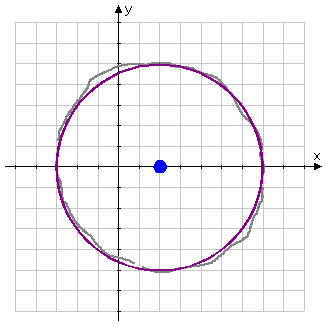It can be very helpful to rotate your paper when you do your drawing, so as to end up with a circle that looks fairly round.

Top  |  1 | 2 | 3  |  Return to Index  Next >>

 Cite this article as: Stapel, Elizabeth. "Conics: Circles: Introduction & Drawing." Purplemath. Available from     https://www.purplemath.com/modules/circle.htm. Accessed [Date] [Month] 2016